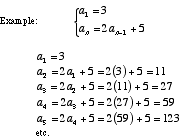# recursive formula of a sequence

Recursive Formula

For a sequencea1, a2, a3, . . . , an, . . . a recursive formula is a formula that requires the computation of all previous terms in order to find the value of an .

Note: Recursion is an example of an iterative procedure.